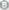﻿ C# winform 连连看游戏 详细教程(适合新手) | Code Bye

# C# winform 连连看游戏 详细教程(适合新手)

7年前 (2015-03-16) 691次浏览
 直接贴上源代码对于菜鸟级新手可以访问我的QQ空间或者微博学习图文并茂的教程（http://user.qzone.qq.com/5747226/infocenter#!app=2&pos=1333162482），如果是有一定C#基础的进阶者可以直接看代码和代码后的注释。如果你是超级菜鸟新手的话你一定会有和我一样的经历与感受。那就是无从入手。市面上的所有的所谓学习宝典与大全其实根本不适合菜鸟举个亲身例子，当我想了解什么是类的概念，翻开大全得到的只是一句简单的名词解释。“类：相同对象的集合”试问什么是对象？什么样的东西又是他娘的集合呢？如果你知道C#的对象和集合概念了你还会不知道什么是类吗？所以说对于菜鸟来说最重要的不是去学习什么类呀什么是封装啊。先从一个十几行、几十行的小程序开始。添加一些控件，玩玩控件的单机事件。写一个银行存储利息计算器，接触学习一下变量的声明和值类型之间的转换。由浅入深的学习。什么样的书是好书，从一个很小的软件开始 穿插一些语法基础知识点 加以详细解释 逻辑指导 图文并茂的展示软件开发的全过程 一个一个开发实例 最后开发一个综合中大型应用程序。这样的书才是好书，但是这样一本书的编写却是很不容易的，而国内就我当时开始学习C#时候这样的书找遍书城只有一本。《C#程序设计-windows项目开发》清华大学出版社的。读完了此书我才有所入门C#winform开发。于是自己萌生了独立写一些小程序的想法并开始网上搜索技术难题，于是找到了这个csdn论坛 ，前些年我在csdn上发过很多求教的帖子，比如上海浮生记，水果机开发等。     好了废话打住，如果你现在和我当时一样状态的话我很愿意分享我的经历给大家和大家一起学习交流下面是源代码的实现 pre class=”brush: C#”> using System; using System.Collections.Generic; using System.ComponentModel; using System.Data; using System.Drawing; using System.Linq; using System.Text; using System.Windows.Forms; using System.Collections;//ArrayList 命名空间 using System.Threading; namespace 连连看 {     public partial class Form1 : Form     {         public Form1()         {             InitializeComponent();         }                 private Bitmap 图集;//各种图案的图片集合                  private int 边长 = 50;//各种图案方块的边长                  private int 尺寸 = 10;//布局大小，行数与列数                  private bool 已选 = false;//布尔值是否已经选中状态                  private int x1, y1, x2, y2;//被选中两个图的坐标                  public Point z1, z2;//折点坐标                  private int 行 = 10, 列 = 10;//行列间距                  private int[] 地图 = new int[12 * 12];//一维数组一百个字节的内存容量                  public sbyte 刷新 = new sbyte();                  private int 已消除 = 25;//用来给以消除的方块赋值-1来记录以消除的方块为哪些         public int a1, a2, b1, b2;//用来消除电脑查找留下的白框 存放临时两点坐标         public sbyte 是否胜利 = 0;//用来储存成功消除队数 日后用来判断是否获胜         public enum 连线方式 { 直连, 一折, 二折 };//枚举三种连线方式         连线方式 啥方式呀=new 连线方式();//实例化一个lxfs         private void 连连看_Load(object sender, EventArgs e)         {                          初始化游戏();             this.CenterToScreen();         }                 private void 初始化游戏()//游戏虚拟地图初始化方法         {             刷新 = 3;             this.pictureBox1.Cursor = Cursors.Hand;             this.pictureBox1.Height = 边长 * (行 + 2);             //设置图片框控件初始高度 由于图形矩阵为10*10 加之上下间隙             //间隙算边长所以上下一共算两个间隙单位                           this.pictureBox1.Width = 边长 * (列 + 2);             this.Height = this.pictureBox1.Height +                 (this.Height – this.ClientRectangle.Height) +                 this.menuStrip1.Height;             /*这里this就是“连连看.Form1”也就是设置窗体的高度 窗体的高度               是根据图片框的高度来定的 所以由图片框高度 加上标题栏和菜单栏               的高度 得出的窗体高度*/                          this.Width = this.pictureBox1.Width +                 (this.Width – this.ClientRectangle.Width);             //窗体宽度基本就是图框宽度了             抽象排列();             生成界面();         }         private void 抽象排列()         {             Random 随机 = new Random();//声明一个随即对象 r             ArrayList 动态数组 = new ArrayList();//声明一个动态数组             for (int i = 0; i < (行 * 列) / 4; i++)             {                 for (int j = 0; j < 4; j++)                 {                     动态数组.Add(i);//动态数组添加4组0到24共计100个ID                 }             }             for (int i = 0; i < (行 * 列); i++)             {                 int nIndex = 随机.Next() % 动态数组.Count;                 地图[i] = (int)动态数组[nIndex];                 动态数组.RemoveAt(nIndex);                 //至此 数组“地图[i] 内字符以 4组 0~24 不规则随机排列”                 //listView1.Items.Add(nIndex.ToString());             }         }                  private void 生成界面()//用被截图排矩阵形成初始化图像         {             Graphics g = 界面();             for (int i = 0; i < 100; i++)//最基本的for循环不解释了             {                                g.DrawImage(截图(地图[i]), 边长 * (i % 尺寸) +                      边长, 边长*(i / 尺寸) + 边长, 边长, 边长);                 //画出10乘10的方图矩阵                 /*由于数组地图[i]已完成4组 0~24 不规则随机排列,故而以“i”                  作为下标来完成截图可以保证所截相同图的ID一致*/                 //Graphics g = this.pictureBox1.CreateGraphics();                 Pen p = new Pen(Color.Black, 3);                 Rectangle a = new Rectangle((i % 尺寸) * 边长 + 边长, (i / 尺寸) * 边长 + 边长, 边长 – 3, 边长 – 3);                 g.DrawRectangle(p, a);             }         }         private Graphics 界面()         //此方法以pictureBox控件的背景作为GDI绘图的画板。方法返回一个Graphics类型         {             if(pictureBox1.Image == null)             {                 Bitmap bmp = new Bitmap(pictureBox1.Width,pictureBox1.Height);                 pictureBox1.Image = bmp;             }             Graphics g = Graphics.FromImage(pictureBox1.Image);             return g;         }                     private Bitmap 截图(int 标号)//截图方法         {             //string 图片路径 = 连连看.Properties.Resources.tp;             //图集 = (Bitmap)Image.FromFile(Application.StartupPath + @”\Image\tp.jpg”);             //图片实例化并且指定图片集合的路径             图集 = 连连看.Properties.Resources.tp;             //这里图集是 Bitmap类型 而资源库里的图片也是此类型可以直接引用                          Bitmap 被截图 = new Bitmap(边长,边长);             //从Bitmap类库中 申明一个 被截图对象 实例化 边长为50                          Graphics 图片 = Graphics.FromImage(被截图);             //GDI绘图 用的是所截图 也就是先前申明的 被截图                                     Rectangle a = new Rectangle(0,0,边长,边长);             //矩形对象 x,y位置 宽高 四个参数                          Rectangle b = new Rectangle(标号 * 64, 0, 64, 64);             /*这里用“标号”来控制所截图的不同，比如当标号=2的时候              这里的截图开始的点就变成78了 就变成第二张图了              前两个也是位置坐标参数 后两个是像素 大小 分别是宽与高              */                          图片.DrawImage(图集,a,b,GraphicsUnit.Pixel);             /*截图方法 图集自然就是要截图的图源               a  指定所绘制图像的位置和大小，将图像进行缩放以适合该矩形。              b  图集对象中要绘制的部分。最后 指定参数所用的度量单位。              总的来说 次方法就把图片一块一块截下来 指定边长为长度的正方形              */             return 被截图;             //方法返回值为bitmap类型 所以返回“被截图”便于之后作为bitmap类             //用于其他方法的参数中         }                  private bool Y直连(int y1, int y2, int x)         {             if (y1 > y2)//判断y坐标大小             {                 int a = y1;                 y1 = y2;                 y2 = a;                 //如果y1小于y2的则两点换位，用一个a来做中介传递参数，应该都看得懂吧             }             //当第一点的y坐标大于第二点y坐标时，第一点在第二点之下             //循环就要用“i = y1 – 1; i > y2; i–”来完成，为了程序的代码可以重复调用             //所以给两点坐标换位来达到第一点y轴坐标永远小于第二点y轴坐标             //这样一来 “i = y1 + 1; i < y2; i++”只要写一次便可以多次调用，             //写代码就简单了 一劳永逸啊。             for (int i = y1 + 1; i < y2; i++)//这里的代码和x直连方法一样，只是方向不同而已             {                 if(i==y2)                 {                     break;                 }                 if(地图[i*10+x]!=已消除)                 {                     return false;                 }             }             return true;         }                  private bool X直连(int x1, int x2,int y)         {             if (x1 > x2)             {                 int n = x1;                 x1 = x2;                 x2 = n;             }             //x轴方法 三个参数 分别是 第一、二两点的x轴坐标和一个y轴坐标，             //因为y轴相同所以y无所谓那个点             for (int i = x1 + 1; i < x2; i++)             {                 //这里循环从第一点的后边一个点开始知道第二点的左边一点                 if (i == x2)//如果两点紧挨着则直接跳出循环                 {                     break;                 }                 if (地图[y * 10 + i] != 已消除)                 //这里用“-1”给数组赋值来记录方块以消除                 //判断数组是否有-1的值也就是说方块是否消                 //除如果没有消除也就无法连通                 {                     return false;//则返回，返回“假”值                 }             }             return true;//不满足以上条件的话则返回“真”值         }         /pre>``` private bool 一折连(int x1, int y1, int x2, int y2)//联通形式为一个折弯的方法         {             if (y1 < y2)             {                 if (地图[y1 * 列 + x2] == 已消除)//右上 ↗                 {                     if (X直连(x1, x2, y1))                     {                         if (Y直连(y1, y2, x2))                         {                             z1.X = x2;                             z1.Y = y1;                             return true;                         }                     }                 }                 if (地图[y2 * 列 + x1] == 已消除)//左下↙                  {                     if (X直连(x1, x2, y2))                     {                         if (Y直连(y1, y2, x1))                         {                             z1.X = x1;                             z1.Y = y2;                             return true;                         }                     }                 }             }             else             {                 if (地图[y2 * 列 + x1] == 已消除)//左上↖                 {                     if (X直连(x1, x2, y2))                     {                         if (Y直连(y1, y2, x1))                         {                             z1.X = x1;                             z1.Y = y2;                             return true;                         }                     }                 }                 if (地图[y1 * 列 + x2] == 已消除)//右下↘                  {                     if (X直连(x1, x2, y1))                     {                         if (Y直连(y1, y2, x2))                         {                             z1.X = x2;                             z1.Y = y1;                             return true;                         }                     }                 }             }             return false;         }         private bool 右边(int x1, int y1, int x2, int y2)//右边 →          {             for (int x = x1 + 1; x <= 列; x++)             {                 if (X直连(x1, x, y1) == true &&                      地图[y1 * 尺寸 + x] == 已消除 &&                      一折连(x, y1, x2, y2) == true)                 {                     z1.X = x;                     z1.Y = y1;                     z2.X = x;                     z2.Y = y2;                     return true;                 }             }             if (X直连(x2, 列, y2))             {                 if (X直连(x1, 列, y1))                 {                     z1.X = 列;                     z1.Y = y1;                     z2.X = 列;                     z2.Y = y2;                     return true;                 }             }             return false;         }                  private bool 左边(int x1, int y1, int x2, int y2)         {             for (int x = 0; x < x1; x++)             {                 if (X直连(x1, x, y1) == true &&                     地图[y1 * 尺寸 + x] == 已消除 &&                     一折连(x, y1, x2, y2) == true)                 {                     z1.X = x;                     z1.Y = y1;                     z2.X = x;                     z2.Y = y2;                     return true;                 }             }             if (X直连(x1, -1, y1))             {                 if (X直连(x2, -1, y2))                 {                     z1.X = -1;                     z1.Y = y1;                     z2.X = -1;                     z2.Y = y2;                     return true;                 }             }             return false;         }                  private bool 上边(int x1, int y1, int x2, int y2)         {             for (int y = 0; y `````` private void 画框(int x, int y, Color 颜色, string 线种)         {             Graphics g = this.pictureBox1.CreateGraphics();//声明图片控件为画板             Pen p = new Pen(颜色, 2);//声明实例化画笔              //p.DashPattern = new float[] { 2, 1 };             if (线种 == "虚线")//如果方法参数写虚线则画笔的参数定义成虚线             {                 p.DashStyle = System.Drawing.Drawing2D.DashStyle.Dot;//定义虚线的样式为点             }             Rectangle a = new Rectangle(x * 边长 - 1 + 边长, y * 边长 - 1 + 边长, 边长 - 2, 边长 - 2);             //画长方形 此方法介绍过多次 这里不再赘述             g.DrawRectangle(p, a);//不为虚线的话直接画实线的         }         private void 画线(int x1,int y1, int x2,int y2,Color 颜色,连线方式 什么方式呢)         {             /*              * 首先画线方法的参数 四个坐标分别是第一点和第二点的x，y坐标              * 接着是颜色 为什么要设个颜色参数呢，和画框一样 画线方法还承担着              * 消除画线的功能 通过再次画和背景颜色一样的线来达到消除白线的目的                         * 最后一个参数就是枚举 是什么连线方式 就如何画线               */             Graphics g = this.pictureBox1.CreateGraphics();//实例化画板没什么说头             Pen p = new Pen(颜色, 2);//实例化一个 “笔”颜色先由变量代替 粗细为 2                         int x_1 = (x1 + 1) * 边长 + 边长 / 2;             //画线是从中间开始二坐标是从左上角开始，所以还是要进行一下加工             //x_1、x_2、y_1、y_1 分别赋值两点的x y 坐标 而z1x、z2x、z1x、z2y             //分别赋值两个折点坐标             int x_2 = (x2 + 1) * 边长 + 边长 / 2;             int y_1 = (y1 + 1) * 边长 + 边长 / 2;             int y_2 = (y2 + 1) * 边长 + 边长 / 2;             int z1x = (z1.X + 1) * 边长 + 边长 / 2;             int z2x = (z2.X + 1) * 边长 + 边长 / 2;             int z1y = (z1.Y + 1) * 边长 + 边长 / 2;             int z2y = (z2.Y + 1) * 边长 + 边长 / 2;                         switch (什么方式呢)//下面用switch循环对号入座 什么方式 什么样的画线             {                 case 连线方式.直连://如果直连的话                     g.DrawLine(p, x_1, y_1, x_2,y_2);//画一条线 参数分别是画笔和四个坐标                     break;//满足条件循环打破                 case 连线方式.一折:                     g.DrawLine(p, x_1, y_1, z1x, z1y);//一折连的话画两条线 第一点到折点                     g.DrawLine(p, z1x, z1y, x_2, y_2);//再从折点到第二点就这么简单                     break;                 case 连线方式.二折:                     g.DrawLine(p, x_1, y_1, z1x, z1y);//二折的话三条线 z1的x，y到z2的                     g.DrawLine(p, z1x, z1y, z2x, z2y);//z2的 x，y 直到x2的x，y                     g.DrawLine(p, z2x, z2y, x_2, y_2);                     break;                 default:                     return;//然则返回             }         }         private void 消除(int x, int y)         {             Graphics g = this.pictureBox1.CreateGraphics();             SolidBrush 刷子 = new SolidBrush(Color.Black);//刷子的声明于实例化 参数为颜色             Rectangle 矩形 = new Rectangle(x*边长+边长,y*边长+边长,边长,边长);             //同样需要一个矩形来规定刷子刷什么样子的图形             g.FillRectangle(刷子,矩形);//然后刷子涂满矩形 把小方块全部涂黑 达到消除方块的目的         }         private bool 相同(int x1, int y1, int x2, int y2)         {             if (地图[y1 * 行 + x1].Equals(地图[y2 * 行 + x2]))             {                 return true;             }             else             {                 return false;             }         }         private void 判断胜利()         {            if(是否胜利.Equals(50))//当是否胜利==50的话 表示100个方块全部消除            {                Form2 f2 = new Form2();//窗体实例化                //这里用 消息框 “MessageBox.show()”来提示也可以。我新建了一个form窗体                //为的是可以自定义提示框 做得新颖一点而已                 f2.ShowDialog();                //显示窗体 这里 f2.Show()就可以显示窗体而 我用了f2.ShowDialog()方法                //为了让用户先处理当前窗体。                this.Hide();//隐藏原窗体 也可以不用隐藏。            }         }          private void pictureBox1_MouseDown(object sender, MouseEventArgs e)         {             if(e.Button==MouseButtons.Right)             {                              }             int x, y;//用来获取鼠标点击后的坐标位置             if (e.Button == MouseButtons.Left)//当鼠标点击的是左键             {                 画框(a1, b1, Color.Black, "实线");//调用画框方法 画实线 黑色达到去除白框                 画框(a2, b2, Color.Black, "实线");                 x = (e.X - 边长) / 边长;//给x赋值                 y = (e.Y - 边长) / 边长;//y同样赋值为鼠标点击后的y轴坐标                 /*格式化鼠标点击的坐标。也就是说，当鼠标点击一个方位得到                 一个X点坐标点，比如 159.86 这时，159.86减去左边留白50得到                 109.86再除以边长50得到的是一个整形值"2" 本来109.86除以50                 应该是2.1978 但是隐形转换过程中 整形值不保留小数点所以得到                 的值为 “2”这样不管鼠标点击的方位是多少，最终得到的是格式化                 的整形数值 0到9                 */                 if (e.X <= 50 || e.Y <= 50 || e.X >= 550 || e.Y >=550)                 {                                          return;                 }                 //这里添加一个判断，当鼠标点击范围超出了游戏界面或者非指定的范围                 //程序返回到初始点，小于50自然是左、上两边留白地方，大于550同样是                 //x、y轴右边与下边界的界外                 if (地图[y * 行 + x] == 已消除)                 {                     return;                 }                 //这里也同样是一个程序异常判断，假如当鼠标点击的y轴为5 x轴为6                 //地图[5乘以10+6]= 地图 则当地图值为-1 表示 点击的地方为                 //空的时候 程序返回。这样不管鼠标点哪里只要碰到空方块就等于没点                 if (已选 == false)                 {                     x1 = x;                     y1 = y;                  //判断是否是第一次选择，因为游戏需要选择两个相同图案的方块才                  //能让程序判断是不是可以消除的图案，所以需要选择两次，当处于                  //“已选值为false”时候把鼠标x轴值赋值给之前声明的整形变量“x1”                  //程序开头已声明此变量《private int x1, y1, x2, y2;//被选中两个图的坐标》                                          画框(x1, y1, Color.White,"实线");                  //给选定的方块画个白色的外框提示玩家 此处调用之前已封装好的“画框”方法                                            已选 = !已选;//布尔值互斥 当值为true时，改值为false 然后反之。                 }                 if (已选 == true)                 {                     x2 = x;//然则把第二次选择的点（与第一次选择的坐标不重复）                     y2 = y;//赋值，把鼠标点击的位置格式化坐标赋值给x2，y2                     //当“已选为true值”时则表示接下来鼠标点击的坐标为第二次选择方块的坐标                     if (x1 == x2 && y1 == y2)//判断当两次点击的位置相同时，第二次选择没有意义                     {                         return;//则返回 重新选择。                     }                     画框(x2, y2, Color.White, "实线");//画框方法                     if (x1 > x2)//如果先点击的坐标点在右边 换位，换位的重要性之前有提到                     {                         int n = x1;                         x1 = x2;                         x2 = n;                         n = y1;                         y1 = y2;                         y2 = n;                     }                     if (相同(x1, y1, x2, y2)==true && 连通判断()==true)                     {                         //如果两点ID 相同 并且 可以联通                         画线(x1, y1, x2, y2, Color.White, 啥方式呀);//画连线 白色                         Thread.Sleep(500);//程序暂停0.5秒                         //Thread类在 using System.Threading;命名空间下 using是引用                         //如果命名空间没有引用的话 System.Threading.Thread.Sleep();亦可                         消除(x1, y1);//方块联通后要消除 调用此方法                         消除(x2, y2);//第二个方块也消除                         画线(x1, y1, x2, y2, Color.Black, 啥方式呀);//消除连线 就是再画条黑色的                         画框(x1, y1, Color.Black, "实线");//画黑框来达到消除白框的目的                         画框(x2, y2, Color.Black, "实线");//第二个方块的黑框                         地图[y1 * 尺寸 + x1] = 已消除;//此坐标点为 以消除状态 赋值25                         地图[y2 * 尺寸 + x2] = 已消除;                         生成界面();//重新生成界面 来达到刷新目的                         已选 = !已选;//布尔值互斥                          是否胜利 += 1;//控制消除方块对数的值 增加一点 表示又多了一对                         判断胜利();//每次连线成功后判断是不是获得胜利                         label1.Text = "已成功消除 " + 是否胜利 + " 对";                     }                                         else//然则                     {                         已选 = false;//已选 布尔值回到初始值                         画框(x1, y1, Color.Black, "实线");//消除画的选框线                         画框(x2, y2, Color.Black, "实线");                         return;//最后返回重新来过                     }                 }             }         }        /pre>``````  private void 退出游戏ToolStripMenuItem_Click(object sender, EventArgs e)         {             Application.Exit();//程序完全关闭的代码         }         private void 帮助ToolStripMenuItem_Click(object sender, EventArgs e)         {            //这里可以自由发挥         }         private void 重新游戏ToolStripMenuItem_Click(object sender, EventArgs e)         {             pictureBox1.Invalidate();//刷新图框控件里的图片数据             抽象排列();//打乱小方图ID             生成界面();//按照打乱的ID 截图并且矩阵排列         }         private void 调整布局ToolStripMenuItem_Click(object sender, EventArgs e)         {             try             {                 刷新--;                 if (刷新.Equals(1))                 {                     //MessageBox.Show("每次游戏只能刷新布局两次","趙趑龍提示：",                     //MessageBoxButtons.OK, MessageBoxIcon.Information);                     //return;                     调整布局ToolStripMenuItem.Enabled = false;                 }                 else                 {                                          pictureBox1.Invalidate();                     Random 随机 = new Random();//声明一个随即对象 r                     ArrayList 动态数组 = new ArrayList();//声明一个动态数组                     for (sbyte i = 0; i < (行 * 列); i++)                     {                         动态数组.Add(地图[i]);                     }                     for (sbyte i = 0; i < (行 * 列); i++)                     {                         int 变换 = 随机.Next() % 动态数组.Count;                         地图[i] = (int)动态数组[变换];                         动态数组.RemoveAt(变换);                     }                     生成界面();                                      }             }             catch (Exception ex)             {                 MessageBox.Show(ex.Message, "趙趑龍提示：",                     MessageBoxButtons.OK, MessageBoxIcon.Information);                              }         }         private void 电脑查找ToolStripMenuItem_Click(object sender, EventArgs e)         {             画框(x1, y1, Color.Black, "实线");             if (电脑查找() == true)             {                 画框(x1, y1, Color.White, "虚线");                 画框(x2, y2, Color.White, "虚线");                 a1 = x1;                 a2 = x2;                 b1 = y1;                 b2 = y2;             }         }            } } /pre>```

CodeBye 版权所有丨如未注明 , 均为原创丨本网站采用BY-NC-SA协议进行授权 , 转载请注明C# winform 连连看游戏 详细教程(适合新手)

[1034331897@qq.com]